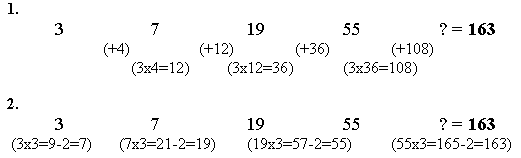#### You may also like### Prompt Cards

These two group activities use mathematical reasoning - one is numerical, one geometric.### Consecutive Numbers

An investigation involving adding and subtracting sets of consecutive numbers. Lots to find out, lots to explore.### Exploring Wild & Wonderful Number Patterns

EWWNP means Exploring Wild and Wonderful Number Patterns Created by Yourself! Investigate what happens if we create number patterns using some simple rules.

# Next Number

##### Age 7 to 11 ShortChallenge Level

Lisa and Alana from Sudbourne School, Lambeth used words to explain how they worked out the next number:

The answer to what is the next number is $163$ because the difference between $3$ and $7$ is $4$ and the next difference (between $7$ and $19$) is $12$ which is $3 \times 4$. The difference after that (between $19$ and $55$) is $36$ which is $12$ (the last difference) times $3$ (the first number, also the number timsed by $4$ to get $12$.) So we just added $108$ to $55$ which equals $163$.

Sinan from IRMAK Primary School, Istanbul, Turkey says much the same thing with numbers:Sarah from Annesley College, Adelaide South Australia explained it a slightly different way:

I figured it out by working out how much the difference is between the four numbers. Then I found that all the numbers between are all factors of three. So, I times each of the between numbers by three. When I got to $55$ times three I got $108$. Then I added $55$ and $108$ and I got $163$. This was the answer I got.

Shivana from Henry Park Primary School, Singapore has another way of writing it:

The answer is $163$: $n \times 3 - 2$ ($n$ being the previous number).

Example $19 \times 3 - 2 = 55$

Emir from IRMAK Primary School, Istanbul, Turkey worked it out in a similar way.

The following people also explained their thinking quite well:

Laura from St Ives School, Haslemere.

Ruyi from Annesley College, Adelaide South Australia

Alex from The Abbey, Woodbridge, Suffolk

Jason from Priory School

Ruth and Jessica from St Lawrence Primary School, Lechlade.

Jenny, Emily, Caroline, Natalie and Rebecca from The Mount School York

Kathryn, Katerina, Nicola and Andrew from Primary Maths Club, International School of Toulouse.

Leyla, Gizem, Unal, Melis, Huma, Ece, Idil, Gizem, Alta, Ece, Simin, Eda S, Ece O, Cana, Sinan, Melike, Efsane, Emre, Ilayda, Baran fromIRMAK Primary School, Istanbul, Turkey.

Thomas and Justin from Tatingstone School

Michael from St Francis Catholic Primary, Essex

Christina from Malborough Primary

And finally, a short neat explanation from Steven from Burpham Primary School, Guildford, Surrey:

I looked at the sequence of numbers and worked out the differences between each one. These were $4$, $12$, $36$.
I realised that $3 \times 4 = 12$, and that $3 \times 12 = 36$, so the next gap would be $3 \times 36$. That is $108$, so the next number is $163$.